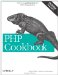# Recipe 4.17. Sorting an Array by a Computable Field

#### 4.17.1. Problem

You want to define your own sorting routine.

#### 4.17.2. Solution

Use usort( ) in combination with a custom comparison function:

`// sort in reverse natural order function natrsort(\$a, \$b) {     return strnatcmp(\$b, \$a); } \$tests = array('test1.php', 'test10.php', 'test11.php', 'test2.php'); usort(\$tests, 'natrsort');`

#### 4.17.3. Discussion

The comparison function must return a value greater that 0 if \$a > \$b, 0 if \$a == \$b, and a value less than 0 if \$a < \$b. To sort in reverse, do the opposite. The function in the Solution, strnatcmp( ), obeys those rules.

To reverse the sort, instead of multiplying the return value of strnatcmp(\$a, \$b) by -1, switch the order of the arguments to strnatcmp(\$b, \$a).

The sort function doesn't need to be a wrapper for an existing sort. For instance, the pc_date_sort( ) function, shown in Example 4-2, shows how to sort dates.

##### pc_date_sort( )

 `// expects dates in the form of "MM/DD/YYYY" function pc_date_sort(\$a, \$b) { list(\$a_month, \$a_day, \$a_year) = explode('/', \$a); list(\$b_month, \$b_day, \$b_year) = explode('/', \$b); if (\$a_year > \$b_year ) return 1; if (\$a_year < \$b_year ) return -1; if (\$a_month > \$b_month) return 1; if (\$a_month < \$b_month) return -1; if (\$a_day > \$b_day ) return 1; if (\$a_day < \$b_day ) return -1; return 0; } \$dates = array('12/14/2000', '08/10/2001', '08/07/1999'); usort(\$dates, 'pc_date_sort');`

While sorting, usort( ) frequently recomputes the sort function's return values each time it's needed to compare two elements, which slows the sort. To avoid unnecessary work, you can cache the comparison values, as shown in pc_array_sort( ) in Example 4-3.

##### pc_array_sort( )

 `function pc_array_sort(\$array, \$map_func, \$sort_func = '') { \$mapped = array_map(\$map_func, \$array); // cache \$map_func() values if ('' == \$sort_func) { asort(\$mapped); // asort() is faster then usort() } else { uasort(\$mapped, \$sort_func); // need to preserve keys } while (list(\$key) = each(\$mapped)) { \$sorted[] = \$array[\$key]; // use sorted keys } return \$sorted; }`

To avoid unnecessary work, pc_array_sort( ) uses a temporary array, \$mapped, to cache the return values. It then sorts \$mapped, using either the default sort order or a user-specified sorting routine. Importantly, it uses a sort that preserves the key/value relationship. By default, it uses asort( ) because asort( ) is faster than uasort( ). (Slowness in uasort( ) is the whole reason for pc_array_sort( ) after all.) Finally, it creates a sorted array, \$sorted, using the sorted keys in \$mapped to index the values in the original array.

For small arrays or simple sort functions, usort( ) is faster, but as the number of computations grows, pc_array_sort( ) surpasses usort( ). The following example sorts elements by their string lengths, a relatively quick custom sort:

`function pc_u_length(\$a, \$b) {     \$a = strlen(\$a);     \$b = strlen(\$b);     if (\$a == \$b) return  0;     if (\$a  > \$b) return  1;                   return -1; } function pc_map_length(\$a) {     return strlen(\$a); } \$tests = array('one', 'two', 'three', 'four', 'five',                'six', 'seven', 'eight', 'nine', 'ten'); // faster for < 5 elements using pc_u_length() usort(\$tests, 'pc_u_length'); // faster for >= 5 elements using pc_map_length() \$tests = pc_array_sort(\$tests, 'pc_map_length');`

Here, pc_array_sort( ) is faster than usort( ) once the array reaches five elements.

Recipe 4.16 for basic sorting and Recipe 4.18 for sorting multiple arrays; documentation on usort( ) at http://www.php.net/usort, asort( ) at http://www.php.net/asort, and array_map( ) at http://www.php.net/array-map .PHP Cookbook: Solutions and Examples for PHP Programmers
ISBN: 0596101015
EAN: 2147483647
Year: 2006
Pages: 445

Similar book on Amazon